# Height and Distance questions for Railway Exams PDF

0
1713

## Height and Distance questions for Railway Exams PDF

Download RRB NTPC Top-15 Height and Distance questions PDF. Questions based on asked questions in previous exam papers very important for the Railway NTPC exam.

Question 1: A ladder 20 m long is leaning against a vertical wall. It makes an angle of 30° with the ground. How high on the wall does the ladder reach?

a) 10 m

b) 17.32 m

c) 34.64 m

d) 30 m

Question 2: A ladder 10 m long is leaning against a vertical wall. It makes an angle of 60° with the ground. How far is the foot of the ladder from the wall?

a) 5 m

b) 8.66 m

c) 17.32 m

d) 15 m

Question 3: The height of a light house is 20 meters above sea level. The angle of depression (from the top of the lighthouse) of a ship in the sea is 30°. What is the distance of the ship from the foot of the light house?

a) 16 m

b) $20\sqrt{3}$

c) 20 m

d) 30 m

Question 4: The angle of elevation of the top of a tower at a distance of 25 m from its foot is 60°. L The approximate height of the tower is

a) 20.3 m

b) 15.3 m

c) 36.3 m

d) 43.3 m

Question 5: From the top of a platform 7 m high, the angle of elevation of a tower was $30^\circ$. If the platform was positioned $50\sqrt{3 }$m away from the tower, how tall was the tower?

a) 57 m

b) 50 m

c) $(25\sqrt{3} + 7)$

d) $25\sqrt{3}$

Question 6: The angle of elevation of the top of a 36 m tall tower from the initial position of a person on the ground was 60°. She walked away in a manner that the foot of the tower, her initial position and the final position wereall in the samestraight line. The angle of elevation of the top of the tower from her final position was 30°. How much did she walk from her initial position?

a) $24\sqrt{3}m$

b) $12\sqrt{3}m$

c) $24m$

d) $36\sqrt{3}m$

Question 7: From an aeroplane just over a straight road, the angles of depression of two consecutive kilometre stones situated at opposite sides of the aeroplane were found to be 60° and 30° respectively. The height (in km) of the aeroplane from the road at that instant was (Given √3 = 1.732)

a) 0.433

b) 8.66

c) 4.33

d) 0.866

Question 8: A person of height 6ft. wants to pluck a fruit which is on a 26/3 ft. high tree. If the person is standing 8/√3 ft. away from the base of the tree, then at what angle should he throw a stone so that it hits the fruit ?

a) 75°

b) 30°

c) 45°

d) 60°

Question 9: The angle of elevation of a tower from a distance of 100 metre from its foot is 30°. Then the height of the tower is

a) $50\sqrt{3}$ metre

b) $100\sqrt{3}$ metre

c) $\frac{50}{\sqrt{3}}$ metre

d) $\frac{100}{\sqrt{3}}$ metre

Question 10: A kite is flying at the height of 75m from the ground. The string makes an angle θ (where cotθ = 8/15) with the level ground. Assuming that there is no slack in the string the length of the string is equal to :

a) 85 metre

b) 65 metre

c) 75 metre

d) 40 metre

Question 11: The angle of elevation of the top of a vertical tower situated perpendicularly on a plane is observed as 60° from a point P on the same plane. From another point Q, 10m vertically above the point P, the angle of depression of the foot of the tower is 30°. The height of the tower is

a) 15 m

b) 30 m

c) 20 m

d) 25 m

Question 12: From a point 20 m away from the foot of a tower, the angle of elevation of the top of the tower is 30°. The height of the tower is

a) 10 √3 m

b) 20 √3 m

c) $\frac{10}{\sqrt{3}}$ m

d) $\frac{20}{\sqrt{3}}$ m

Question 13: The shadow of a tower standing on a level ground is found to be 40 metre longer when the suns altitude is 30° than when it is 60°. Find the length of the tower,

a) 20√3 m

b) 10 m

c) 10√3 m

d) 20 m

Question 14: The length of the shadow of a tower is 9 metres when the sun’s altitude is 30°. What is the height of the tower ?

a) $3\sqrt{3}$ m

b) $4\frac{1}{2}$ m

c) $9\sqrt{3}$ m

d) $\frac{9\sqrt{3}}{2}$ m

Question 15: From the top of a cliff 90 metre high, the angles of depression of the top and bottom of a tower are observed to be 30° and 60° respectively. The height of the tower is :

a) 45 m

b) 60 m

c) 75 m

d) 30 m

From the given information, Below is the figure formed.

From the figure,We can say $Sin 30 = \frac{height of wall}{height of ladder}$

=> $\frac{1}{2}=\frac{height of wall}{20}$

=> height of wall = 10m

Let say,height of the wall is ‘a’ and

distance between the foot of the wall and foot of the ladder is ‘b’.

Given that ,height of the ladder is 10 m.

So,$a^2+b^2=10^2$

or,$a^2+b^2=100$……….(1)

And angle between the ladder and ground is 60°.

so, $tan\theta=tan60°=√3.$

Or,$√3=(a/b).$

or,$a=√3b.$

put this value in equation (1):

$(√3b)^2+b^2=100$

or,$4b^2=100$

or,$b^2=25$

or,b=5.(-5 can not be the value of distance)

So,a=5√3.

So,the ladder is 5 m away from the base of the wall.

A is correct choice.

angle of depression = 30° = angle of elevation from the boat

height of light house = 20m

let the distance btw the light house and boat be x

tanФ= $\frac{opp}{adj}$

tan30°= $20\div \text{distance btw the light house and boat}$

$\dfrac{1}{\sqrt{3}}=20\div x$

x=$20\sqrt{3}$

tan 60 = $\sqrt{3}$ = $\frac{h}{25}$

=> h = 25$\sqrt{3}$

=> 43.3 m

In triangle ABC BC = DE = $50\sqrt{3}$, BD = 7 m

$\tan30^{\circ\ }=\frac{AC}{BC}=\frac{AC}{50\sqrt{3}}$

$\frac{1}{\sqrt{\ 3}}=\frac{AC}{50\sqrt{3}}$

$AC=50\ m$

Length of the tower = 50 + 7 = 57 m

Let us consider CD as x

From the fig, consider

tan 60 = $\frac{AB}{BC}$

tan 60 = $\frac{AB}{BC}$ = $\sqrt{3}$ =  $\frac{36}{BC}$

By simplifying

BC = $12\times \sqrt{3}$

tan 30 = $\frac{36}{BC + x}$ = $\frac {1}{\sqrt{3}}$ =   $\frac {36}{12\sqrt{3+ x}}$

By simplifying

= $24\sqrt{3}m$ = 41.568 m.

She walked nearly 41.568 m.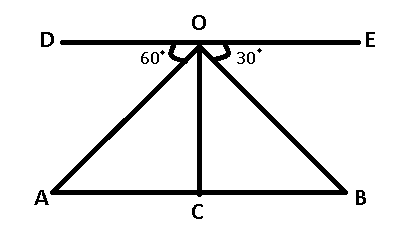OC = height of plane = $h$

$\angle$OAC = $\angle$DOA = 60°

$\angle$OBC = $\angle$BOE = 30°

AB = 2 and let AC = $x$

=> BC = $(2-x)$

From, $\triangle$OAC

$tan60^{\circ} = \frac{OC}{AC}$

=> $\sqrt{3} = \frac{h}{x}$

=> $x = \frac{h}{\sqrt{3}}$ ————Eqn(1)

From, $\triangle$OBC

$tan30^{\circ} = \frac{OC}{BC}$

=> $\frac{1}{\sqrt{3}} = \frac{h}{2-x}$

=> $\sqrt{3}h = 2 – \frac{h}{\sqrt{3}}$ [From eqn(1)]

=> $\frac{3h+h}{\sqrt{3}} = 2$

=> $h = \frac{2\sqrt{3}}{4} = \frac{\sqrt{3}}{2}$

= $\frac{1.732}{2}$ = 0.866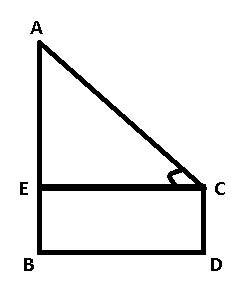Height of person = CD = 6 ft

Height of tree = AB = $\frac{26}{3}$ ft

Distance between them = BD = $\frac{8}{\sqrt{3}}$ ft

To find : $\angle$ACE = $\theta$ = ?

Solution : AE = AB – BE = $\frac{26}{3}$ – 6

=> AE = $\frac{8}{3} ft$

and BD = CE = $\frac{8}{\sqrt{3}}$ ft

Now, in $\triangle$AEC

=> $tan\theta$ = $\frac{AE}{CE}$

=> $tan\theta$ = $\frac{\frac{8}{3}}{\frac{8}{\sqrt{3}}}$

=> $tan\theta$ = $\frac{1}{\sqrt{3}}$

=> $\theta$ = 30°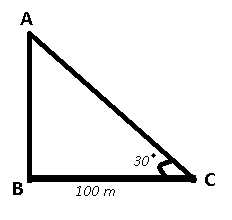Height of tower = AB

In $\triangle$ABC

=> $tan\theta = \frac{AB}{BC}$

=> $tan30^{\circ} = \frac{AB}{100}$

=> $\frac{1}{\sqrt{3}} = \frac{AB}{100}$

=> $AB = \frac{100}{\sqrt{3}}$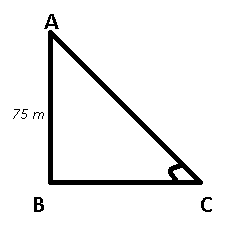Height of kite from ground = AB = 75 m

$\angle$ACB = $\theta$

We know that $cot\theta = \frac{8}{15}$

=> $\frac{BC}{AB} = \frac{8}{15}$

=> $BC = \frac{8*75}{15} = 40 m$

Now, length of string AC = $\sqrt{(AB)^2 + (BC)^2}$

=> AC = $\sqrt{75^2 + 40^2}$

= $\sqrt{5625+1600} = \sqrt{7225}$

=> AC = 85 m

In the given problem, distance between tower and point of observation will be the adjacent side and tower will be the opposite side.
Let ‘d’ be the distance and ‘h’ be the height of the tower.
tan 60 = h/d => d = h/tan 30
tan 30 = (h-10)/d => d = (h-10)/ tan 60
h/tan 30 = (h-10)/tan 60
h/h-10 = 3
2h = 30
h = 15m
Option A is the correct answer.

Perpendicular AB= height of tower

Base BC = distance b/w base of tower and the point

Hypotenuse AC= segment b/w top of the tower and the point

Angle of elevation ACB =30°

tan 30° = Perpendicular / Base = 1/√3

Perpendicular = Base*1/√3

Base is 20 m.

So height of tower is 20/√3m.

We know that, length of shadow will be equal to the adjacent side and the height of tower is the opposite side of the triangle.
We have been given that difference in lengths of shadows = 40m.
Length of shadow = h tan A.
h tan 60 – h tan 30 = 40
h√3 – h/√3 = 40
(3h -h)/√3 = 40
2h = 40√3
h = 20√3 m.
Option A is the right answer.

Height of tower will be the opposite side.
Hence, tan 30 = height/length
$1/\sqrt{3}$ = $h/9$
=>$h = 3\sqrt{3}$
Option A is the right answer.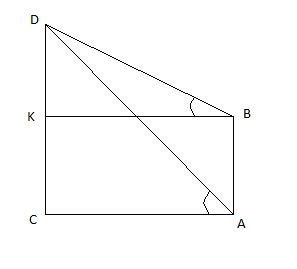Given: CD = 90 meter;
∠KBD = 30° & ∠CAD = 60°
BD × cos30° = BK
BD × sin30° = DK
BK = AC
BD × cos30° = AD × cos60°
√ 3 × BD = AD ____________ (1)
Now, DC = DK + KC
DC – DK = AB [As, KC = AB]
(AD × sin60°) – (BD × sin30°) = AB
Using equation (1)
($\sqrt{3}$ ×BD×$\frac{\sqrt{3}}{2}$)BD2=AB

(3×BD×32)−BD2=AB

AB = BD _____________ (2)
Now, AD × sin 60° = DC = 90 meter
AD=$\frac{180}{\sqrt{3}}$

From (1)
BD=AD/$\sqrt{3}$

=180/$\sqrt{3} \times \sqrt{3}$ = 60 meter

From (2) AB = 60 meter
Option B is the correct answer.

We hope this Top-15 Height and Distance questions pdf for RRB NTPC exam will be highly useful for your Preparation.